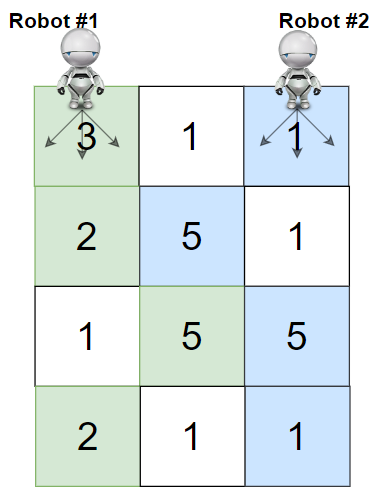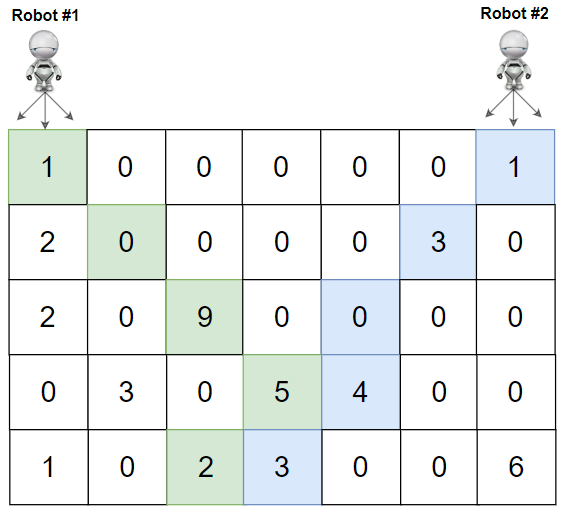Formatted question description: https://leetcode.ca/all/1463.html

# 1463. Cherry Pickup II (Hard)

Given a rows x cols matrix grid representing a field of cherries. Each cell in grid represents the number of cherries that you can collect.

You have two robots that can collect cherries for you, Robot #1 is located at the top-left corner (0,0) , and Robot #2 is located at the top-right corner (0, cols-1) of the grid.

Return the maximum number of cherries collection using both robots  by following the rules below:

• From a cell (i,j), robots can move to cell (i+1, j-1) , (i+1, j) or (i+1, j+1).
• When any robot is passing through a cell, It picks it up all cherries, and the cell becomes an empty cell (0).
• When both robots stay on the same cell, only one of them takes the cherries.
• Both robots cannot move outside of the grid at any moment.
• Both robots should reach the bottom row in the grid.

Example 1:Input: grid = [[3,1,1],[2,5,1],[1,5,5],[2,1,1]]
Output: 24
Explanation: Path of robot #1 and #2 are described in color green and blue respectively.
Cherries taken by Robot #1, (3 + 2 + 5 + 2) = 12.
Cherries taken by Robot #2, (1 + 5 + 5 + 1) = 12.
Total of cherries: 12 + 12 = 24.


Example 2:Input: grid = [[1,0,0,0,0,0,1],[2,0,0,0,0,3,0],[2,0,9,0,0,0,0],[0,3,0,5,4,0,0],[1,0,2,3,0,0,6]]
Output: 28
Explanation: Path of robot #1 and #2 are described in color green and blue respectively.
Cherries taken by Robot #1, (1 + 9 + 5 + 2) = 17.
Cherries taken by Robot #2, (1 + 3 + 4 + 3) = 11.
Total of cherries: 17 + 11 = 28.


Example 3:

Input: grid = [[1,0,0,3],[0,0,0,3],[0,0,3,3],[9,0,3,3]]
Output: 22


Example 4:

Input: grid = [[1,1],[1,1]]
Output: 4


Constraints:

• rows == grid.length
• cols == grid[i].length
• 2 <= rows, cols <= 70
• 0 <= grid[i][j] <= 100

Related Topics:
Dynamic Programming

## Solution 1. DP Top-down

It’s a typical DP problem. The best answer for a given row index i, and column index a for the robot #1 and b for the robot #2 is fixed, but there are multiple ways to get to this state i, a, b, so we can use dp to memorize the corresponding answer.

Let dp(i, a, b) be the max number of cherries we can get given state i, a, b.

dp(i, a, b) = sum( dp(i + 1, p, q) | (i+1, p), (i+1, q) are the points reachable from (i, a) and (i, b) )


Since there are some states unreacheable at the upper part of the grid, using top-down DP can easily skip the computation for those states.

// OJ: https://leetcode.com/problems/cherry-pickup-ii/

// Time: O(M * N^2)
// Space: O(M * N^2)
class Solution {
int m, M, N;
int dp(vector<vector<int>> & A, int i, int a, int b) {
if (i == M) return 0;
if (m[i][a][b] != -1) return m[i][a][b];
int v = a == b ? A[i][a] : (A[i][a] + A[i][b]);
int ans = 0;
for (int x = -1; x <= 1; ++x) {
for (int y = -1; y <= 1; ++y) {
int p = a + x, q = b + y;
if (p < 0 || q < 0 || p >= N || q >= N) continue;
ans = max(ans, dp(A, i + 1, p, q));
}
}
return m[i][a][b] = v + ans;
}
public:
int cherryPickup(vector<vector<int>>& A) {
M = A.size(), N = A.size();
memset(m, -1, sizeof(m));
return dp(A, 0, 0, N - 1);
}
};


## Solution 2. DP Bottom-up

Populate from the last row to the first row.

The rth row pulls values from the r + 1th row.

// OJ: https://leetcode.com/problems/cherry-pickup-ii/

// Time: O(M * N^2)
// Space: O(M * N^2)
class Solution {
public:
int cherryPickup(vector<vector<int>>& A) {
int M = A.size(), N = A.size(), dp = {0};
for (int r = M - 1; r >= 0; --r) {
for (int i = 0; i < N; ++i) {
for (int j = i + 1; j < N; ++j) {
for (int a = i - 1; a <= i + 1; ++a) {
if (a < 0 || a >= N) continue;
for (int b = j - 1; b <= j + 1; ++b) {
if (b < 0 || b >= N || b <= a) continue;
dp[r][a][b] = max(dp[r][a][b],
(a == b ? A[r][a] : (A[r][a] + A[r][b])) + dp[r + 1][i][j]);
}
}
}
}
}
return dp[N - 1];
}
};


## Solution 3. DP Bottom-up

Populate from the last row to the first row.

The rth row pushes values to the r - 1th row.

// OJ: https://leetcode.com/problems/cherry-pickup-ii/

// Time: O(M * N^2)
// Space: O(M * N^2)
class Solution {
public:
int cherryPickup(vector<vector<int>>& A) {
int M = A.size(), N = A.size(), dp = {0};
for (int r = M - 1; r > 0; --r) {
for (int i = 0; i < N; ++i) {
for (int j = i + 1; j < N; ++j) {
dp[r][i][j] += i == j ? A[r][i] : A[r][i] + A[r][j];
for (int a = i - 1; a <= i + 1; ++a) {
if (a < 0 || a >= N) continue;
for (int b = j - 1; b <= j + 1; ++b) {
if (b < 0 || b >= N || b <= a) continue;
dp[r - 1][a][b] = max(dp[r - 1][a][b], dp[r][i][j]);
}
}
}
}
}
return dp[N - 1] + A + A[N - 1];
}
};


## Solution 4. DP Bottom-up

Populate from the first row to the last row.

The rth row pulls values from r - 1th row.

If the previous state is not reachable dp[r - 1][i][j] == -1, we don’t need to pull.

// OJ: https://leetcode.com/problems/cherry-pickup-ii/

// Time: O(M * N^2)
// Space: O(M * N^2)
class Solution {
public:
int cherryPickup(vector<vector<int>>& A) {
int M = A.size(), N = A.size(), dp, ans = 0;
memset(dp, -1, sizeof(dp));
dp[N - 1] = A + A[N - 1];
for (int r = 1; r < M; ++r) {
for (int i = 0; i < N; ++i) {
for (int j = i + 1; j < N; ++j) {
if (dp[r - 1][i][j] == -1) continue;
for (int a = i - 1; a <= i + 1; ++a) {
if (a < 0 || a >= N) continue;
for (int b = j - 1; b <= j + 1; ++b) {
if (b < 0 || b >= N || b <= a) continue;
dp[r][a][b] = max(dp[r][a][b],
dp[r - 1][i][j] + (a == b ? A[r][a] : A[r][a] + A[r][b]));
if (r == M - 1) ans = max(ans, dp[r][a][b]);
}
}
}
}
}
return ans;
}
};


## Solution 4. DP Bottom-up

Populate from the first row to the last row.

The rth row pushes values to the r + 1th row.

If the current state is not reachable dp[r][i][j] == -1, we don’t need to push.

// OJ: https://leetcode.com/problems/cherry-pickup-ii/

// Time: O(M * N^2)
// Space: O(M * N^2)
class Solution {
public:
int cherryPickup(vector<vector<int>>& A) {
int M = A.size(), N = A.size(), dp, ans = 0;
memset(dp, -1, sizeof(dp));
dp[N - 1] = A + A[N - 1];
for (int r = 0; r < M - 1; ++r) {
for (int i = 0; i < N; ++i) {
for (int j = i + 1; j < N; ++j) {
if (dp[r][i][j] == -1) continue;
for (int a = i - 1; a <= i + 1; ++a) {
if (a < 0 || a >= N) continue;
for (int b = j - 1; b <= j + 1; ++b) {
if (b < 0 || b >= N || b <= a) continue;
dp[r + 1][a][b] = max(dp[r + 1][a][b],
dp[r][i][j] + (a == b ? A[r + 1][a] : A[r + 1][a] + A[r + 1][b]));
if (r + 1 == M - 1) ans = max(ans, dp[r + 1][a][b]);
}
}
}
}
}
return ans;
}
};


## Solution 5. DP Bottom-up

Since we only care about the recent two rows, we can reduce the dp array size from M * N^2 to N^2 by swapping arrays.

In the following code, we are populating from the first row to the last row, and the rth row pulls values from r - 1th row.

// OJ: https://leetcode.com/problems/cherry-pickup-ii/

// Time: O(M * N^2)
// Space: O(N^2)
class Solution {
public:
int cherryPickup(vector<vector<int>>& A) {
int M = A.size(), N = A.size(), ans = 0;
vector<vector<int>> d(N, vector<int>(N, -1)), e(N, vector<int>(N));
d[N - 1] = A + A[N - 1];
for (int i = 1; i < M; ++i) {
for (int j = 0; j < N; ++j) {
for (int k = j + 1; k < N; ++k) e[j][k] = -1;
}
for (int j = 0; j < N; ++j) {
for (int k = j + 1; k < N; ++k) {
if (d[j][k] < 0) continue;
for (int a = j - 1; a <= j + 1; ++a) {
if (a < 0 || a >= N) continue;
for (int b = k - 1; b <= k + 1; ++b) {
if (b < 0 || b >= N) continue;
e[a][b] = max(e[a][b], d[j][k] + (a == b ? A[i][a] : (A[i][a] + A[i][b])));
}
}
}
}
swap(d, e);
}
for (int j = 0; j < N; ++j) {
for (int k = j + 1; k < N; ++k) ans = max(ans, d[j][k]);
}
return ans;
}
};


Java

class Solution {
public int cherryPickup(int[][] grid) {
int rows = grid.length, columns = grid.length;
Set<Integer> visitedSet = new HashSet<Integer>();
Queue<int[]> queue = new LinkedList<int[]>();
queue.offer(new int[]{columns - 1, grid + grid[columns - 1]});
for (int i = 1; i < rows; i++) {
Map<Integer, Integer> map = new HashMap<Integer, Integer>();
int size = queue.size();
for (int j = 0; j < size; j++) {
int[] array = queue.poll();
int positions = array, cherries = array;
int position0 = positions / columns, position1 = positions % columns;
for (int k = position0 - 1; k <= position0 + 1; k++) {
if (k < 0 || k >= columns)
continue;
for (int m = Math.max(k + 1, position1 - 1); m <= position1 + 1; m++) {
if (m < 0 || m >= columns)
continue;
int newPosition = k * columns + m;
int newCherries = k == m ? cherries + grid[i][k] : cherries + grid[i][k] + grid[i][m];
int max = Math.max(map.getOrDefault(newPosition, 0), newCherries);
map.put(newPosition, max);
}
}
}
Set<Integer> keySet = map.keySet();
for (int newPosition : keySet)
queue.offer(new int[]{newPosition, map.get(newPosition)});
}
int maxCherries = 0;
while (!queue.isEmpty()) {
int[] array = queue.poll();
maxCherries = Math.max(maxCherries, array);
}
return maxCherries;
}
}